# Enum and Typedef in C++

## Enum and Typedef in C++ with Examples:

In this article, I am going to discuss Enum which is an enumerated data type, and Typedef in C++ with Examples. Please read our previous article where we discussed Bitwise Operators in C++ with Examples. At the end of this article, you will understand everything about Enum and Typedef in C++ with Examples.

##### Enum in C++:

Enum is useful for defining user-defined data types. As a programmer, we can define our own data types. There are a lot of data types given in C++ like integer, float, double, and so on. If we want to define our own data type then we can define but we cannot introduce something new. We have to use the existing data types only and define them.

##### Where do we use Enum in C++?

Let us see examples where we can use an enum. For a better understanding, please have a look at the below image.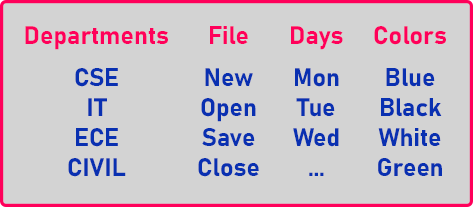1st example is ‘Department’, we can use these departments in our application like a student belongs to this department. How do we want to store information of employees or faculty belonging to which department? For that we will write the name of the department means it will be a string.

Now, working with string is a little time-consuming process because it’s not one letter, it’s a set of characters. So, we want to make it faster. Mostly in daily life, we find that if we have some commonly used terms or words, we define codes for them.

So, we define ‘codes’ in programming this is very common that if you have a limited set of words commonly used, then you can assign codes for them and you can use those codes for that word. So, In the ‘Departments’, we can give codes like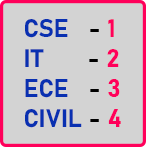In this way, we assigned codes for each word. So, we can use numbers instead of using strings. This will be a faster and easy way. Similarly, we have other examples. Also, let us look at them.This is the file menu in any application. If you open any application, then you will have some options but here we have taken only a few options. Here ‘0’ means ‘New’, ‘1’ means ‘Open’, ‘2’ means ‘Save’ and ‘3’ means ‘Close’.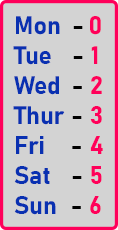We can give the codes similarly here. Here we have assigned codes for the day’s name. So numeric values will represent these words. These were the examples. Now we want to learn how we can define these codes in our programs and how we can use them in C++. There is more than one method for doing this.

1st Method of Defining Constant in C++: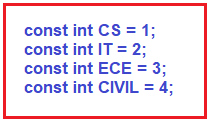We can define constants and store values in these constants. So, if there are 4 then we can define 4 constants, and if there are 10 then we can define 10 such constants in our application. Wherever we write ‘CS’ in our program, then it means ‘1’ because we store 1 value in the ‘CS’ constant. This is the 1st method of defining Enum in the C++ Language. If we want to define more than 10 or 100 codes then this would be too lengthy. So, in that case, we can follow the second method which is given below.

##### 2nd method of Defining Constant in C++:

enum day {mon, tue, wed, thur, fri, sat, sun};

So, this is another way to collectively define the codes together. This is enum. Here we have used the ‘enum’ key to define all of the codes together. Here, ‘mon’ will be ‘0’, ‘tue’ will be ‘1’, ‘wed’ will be ‘2’, and so on. Automatically they will take all these values. So instead of writing them constants one by one, we have written them with the help of ‘enum’.

Now ‘day’ is a new data type. This is the user-defined data type, and it has values from Monday to Sunday. The value of Monday is ‘0’, so automatically it assigns values to others with one incremented as the before value. So, it defines the collection of values. We don’t have to mention 0, 1, 2, 3… By default, the first one is ‘0’s

##### Enum Pseudocode: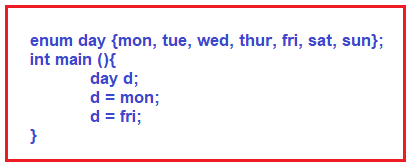We have declared the variable of type ‘day’. ‘d’ is the variable of type ‘day’. It’s a data type that we have defined outside the main function.

##### Now, what are the values that they can have?

We can assign anything from a set of values that we have defined outside the main function i.e. mon, tue, etc. From those sets of values, we can assign anything but we cannot directly assign the value to any integer i.e. d = 0.

So, this will be invalid. You have to use those sets of values only at any place in your program. We can check any values like if (d == mon). If ‘d’ is equal to Monday then the answer will be true and it will enter into this if block.

##### Department Example using Enum

Let us define the enum of the Department example.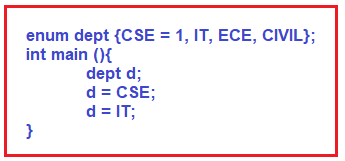If we don’t want the starting value as 0 then we can assign it to other values as we did in the above example. Then from that value, the rest of the value will be assigned accordingly i.e. CSE = 1 then IT will be 2, ECE will be 3, and so on. So, if we mentioned some starting number then the remaining will take the next number automatically.

Now in our program, inside the main function, we have declared a variable of type Department ‘dept’. Then ‘d’ assign CSE, you can also assign any other department, so you can declare the variable which can have only this set of values. You cannot write anything other than this.

##### Another Example:

enum day {mon=1, tue, wed=4, thur, fri, sat=9, sun};

Here we have written ‘mon = 1’, then automatically ‘tue’ will 2, next we assigned ‘wed’ as 4, then by default ‘thur’ will be 5 and ‘fri’ will be 6, next we assigned ‘sat’ as 9 then ‘sun’ will be 10. So, we can mention the values in different places like the above example.

We can change the values but the next term or the next constant will be the next number. So, this is about the enum. By using this, the program becomes more readable and easier for programming. Now next is Typedef.

##### Complete Enum Example Code in C++ Language:
```#include <iostream>
using namespace std;

enum day {mon=1, tue, wed, thu=4, fri, sat=9, sun};
enum dept {CS=1, IT, ECE, CIVIL, MECH, AERO};

int main(){
day d1, d2;
dept dt1, dt2;

d1 = mon;
d2 = thu;

dt1 = CIVIL;
dt2 = ECE;

cout << "day 1: " << d1 << " & day 2: " << d2 << endl;
cout << "department 1: " << dt1 << " & ddepartment 2: " << dt2 << endl;

return 0;
}
```
###### Output: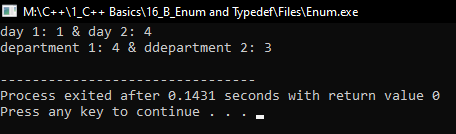##### Typedef in C++:

Let us look at typedef that is ‘Type Definition’. So, for the explanation, we have taken one example here.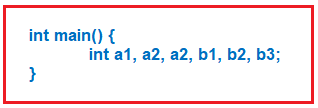Here we have some variables. All these are of type Integer and variable names are a1, a2, b1, b2, b3. We have not used meaningful names or readable names. Usually, programmers have the habit of doing this. For quickly they will give the names like this. They will not give lengthy names or more meaningful names so that will become their habit. So even in your real projects or the major projects also you will follow the same thing.

If the project is becoming Lengthy and is taking much time it is very difficult for you to work on the project because you yourself cannot figure out what is a1, a2, b1, b2, etc. So, this type of problem arises if you’re not using a readable or meaningful name. This can create a readability issue.

We want to make those variables meaningful or if not the names, then at least data types should tell me about the variables. So yes, that we can do by using typedef. Let us do it.

int a1, a2, a3, b1, b2, b3; In this statement, a1, a2, a3 are price and b1, b2, b3 are quantity.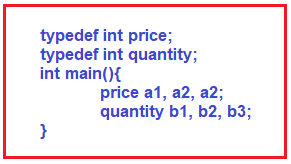Here we have defined typedef. This is a type definition integer for price and quantity. Inside main, now it is easy to read the data because here the data type tells us about the variable. Here a1, a2, a3 represent prices, and b1, b2, b3 represent quantity.

Typedef is useful for defining user-defined data types. Here we have our own data type now. This is nothing but the change of the name of an integer. We are calling it price, price is an integer. So instead of giving documentation or giving lengthy names, you can use a typedef.

##### Complete Typedef Example Code in C++ Language:
```#include <iostream>
using namespace std;

typedef int price;
typedef int quantity;

int main(){
price a1, a2;
quantity b1, b2;

a1 = 39;
a2 = 75;

b1 = 6;
b2 = 3;

cout << "prices are: " << a1 << " " << a2 << endl;
cout << "quantity are: " << b1 << " " << b2 << endl;

return 0;
}
```
###### Output: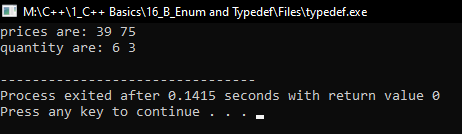In the next article, I am going to discuss Conditional Statements in C++ with Examples. Here, in this article, I try to explain Enum and Typedef in C++ with Examples and I hope you enjoy this Enum and Typedef in C++ with Examples article.